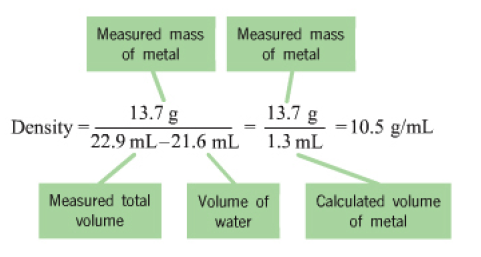###### FREE Expert SolutionView Complete Written Solution
###### Problem Details

Part A. To save time you can approximate the initial volume of water to ±1 mL and the initial mass of the solid to ±1 g . For example, if you are asked to add 23 mL of water, add between 22 mL and 24 mL.

Which metals in each of the following sets will have equal density?
Check all that apply.

a. 20.2 g silver placed in 21.6 mL of water and 20.2 g copper placed in 21.6 mL of water.

b. 15.4 g gold placed in 20.0 mL of water and 15.7 g silver placed in 20.0 mL of water.

c. 20.2 g silver placed in 21.6 mL of water and 12.0 g silver placed in 21.6 mL of water.

d. 20.2 g gold placed in 21.6 mL of water and 12.0 g copper placed in 21.6 mL of water.

e. 11.2 g gold placed in 21.6 mL of water and 14.9 g gold placed in 23.4 mL of water.

f. 15.2 g copper placed in 21.6 mL of water and 50.0 g copper placed in 23.4 mL of water.

The density of a substance is an intrinsic property and is independent of the amount of substance present. It is often used to identify a substance.

Water displacement can be used to find the volume of a solid by placing the object in a known volume of water. When the object is added to water, the measured total volume increases. This final volume is equal to the volume of the initial water plus the volume of the solid object added. To determine the volume of the water that is displaced by the object subtract the initial volume from the final volume. Using a scale to measure the mass of the object, you can now calculate the density of the object as its mass divided by its volume.

For example, if you add 13.7 g of silver to 20.0 mL of water, the final volume observed in the measuring cylinder is 21.3 mL. The change in volume is 21.3 mL − 20.0 mL = 1.3 mL. The density of silver is thus

Each calculation is shown after clicking to calculate the density in the simulationFollow the steps provided in the simulation to add water to the graduated cylinder, select one of the three samples (copper, silver, or gold), set its mass to the values given in the statements below, and calculate its density. Here is a summary of the steps required:

1. Prepare a known volume of water until the desired volume of water has been added. If more than the desired volume is added.

2. Weigh out metal until the desired amount of metal is added to the weighing pan.

3. Calculate density to see how the density is calculated using water displacement to measure the volume of the solid.